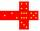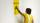# Cube 5

The content area of one cube wall is 32 square centimeters. Determine the length of its edges, its surface and volume.

Result

a =  5.657 cm
S =  192 cm2
V =  181.024 cm3

#### Solution:Leave us a comment of example and its solution (i.e. if it is still somewhat unclear...):Be the first to comment!#### To solve this example are needed these knowledge from mathematics:

Tip: Our volume units converter will help you with converion of volume units.

## Next similar examples:

1. Cube surface areaWall of cube has content area 99 cm square. What is the surface of the cube?
2. The cubeThe surface of the cube is 150 square centimeters. Calculate: a- the content of its walls b - the length of its edges
3. Cube wallsFind the volume and the surface area of the cube if the area of one of its walls is 40 cm2.
4. Cube edgesThe sum of the lengths of the cube edges is 42 cm. Calculate the surface of the cube.
5. Edges or sidesCalculate the cube volume, if the sum of the lengths of all sides is 276 cm.
6. Cube cornersThe wooden cube with edge 64 cm was cut in 3 corners of cube with edge 4 cm. How many cubes of edge 4 cm can be even cut?
7. Volume of cubeSolve the volume of a cube with width 26cm .
8. The numberThe number of 1 cm cubes required to make 4 cm cube is?
9. Wall paintingThe wall is 5 meters wide and 2 meters high. Window in the wall has dimensions 2x1,8 meters. How many litera of color is needed to paint two-layer this walls, if the 1 m2 needs 1.1 liters of paint?
10. Cuboid - VabFind the surface of the cuboid when its volume is 52.8 cubic centimeters, and the length of its two edges is 2 centimeters and 6 centimeters.
11. Cuboid surfaceDetermine surface area of cuboid if its volume is 52.8 cm cubic and length of the two edges are 2 cm and 6 cm.
12. Surface of cuboidFind the surface of the cuboid if its volume is 52.8 cm3 and the length of its two edges is 2 cm and 6 cm.
13. CuboidCuboid has a surface of 516 cm2. Side a = 6 cm and b = 12 cm. How long is the side c =?
14. AlleyAlley measured a meters. At the beginning and end are planted poplar. How many we must plant poplars to get the distance between the poplars 15 meters?
15. SimplifySimplify the following problem and express as a decimal: 5.68-[5-(2.69+5.65-3.89) /0.5]
16. ExpressionSolve for a specified variable: P=a+4b+3c, for a
17. HotelThe hotel has a p floors each floor has i rooms from which the third are single and the others are double. Represents the number of beds in hotel.